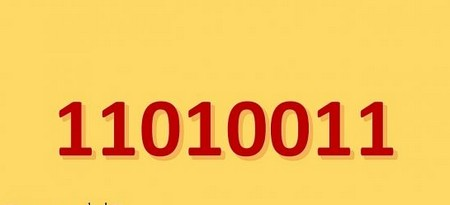##Number conversion and binary artihmeticVideo: .MP4, 1920x1080, 30 fps | Audio: AAC, 48 kHz, 2ch | Duration: 2h 8m
Genre: eLearning | Language: English | Size: 544 MB

Number converter : .

This is a course about numbers conversion and binary arithmetic, it contains 44 lectures for a total of 2 hours
ly/numberConverter
Why would you take this course :
- Simple explications
- A lot of examples/practice
- By taking this course, you will be able to :
1. Understand the concept of number systems
2. Convert from/to decimal, binary, octal, and hexadecimal system quickly
3. Convert from/to IEEE 754, BCD, ASCII and gray quickly
4. Solve binary arithmetic operations (addition/subtraction/multiplication/division) quickly